## Selection Sort-

• Selection sort is one of the easiest approaches to sorting.
• It is inspired from the way in which we sort things out in day to day life.
• It is an in-place sorting algorithm because it uses no auxiliary data structures while sorting.

## How Selection Sort Works?

Consider the following elements are to be sorted in ascending order using selection sort-

6, 2, 11, 7, 5

Selection sort works as-

• It finds the first smallest element (2).
• It swaps it with the first element of the unordered list.
• It finds the second smallest element (5).
• It swaps it with the second element of the unordered list.
• Similarly, it continues to sort the given elements.

As a result, sorted elements in ascending order are-

2, 5, 6, 7, 11

## Selection Sort Algorithm-

Let A be an array with n elements. Then, selection sort algorithm used for sorting is as follows-

```for (i = 0 ; i < n-1 ; i++)
{
index = i;
for(j = i+1 ; j < n ; j++)
{
if(A[j] < A[index])
index = j;
}
temp = A[i];
A[i] = A[index];
A[index] = temp;
}```

Here,

• i = variable to traverse the array A
• index = variable to store the index of minimum element
• j = variable to traverse the unsorted sub-array
• temp = temporary variable used for swapping

## Selection Sort Example-

Consider the following elements are to be sorted in ascending order-

6, 2, 11, 7, 5

The above selection sort algorithm works as illustrated below-

### Step-01: For i = 0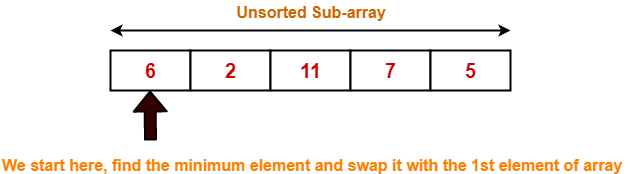### Step-02: For i = 1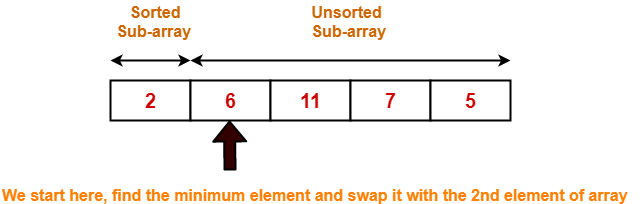### Step-03: For i = 2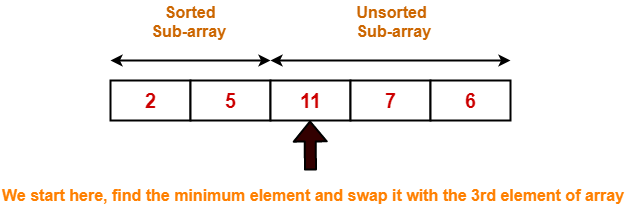### Step-04: For i = 3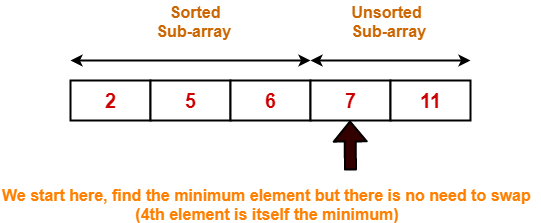### Step-05: For i = 4

Loop gets terminated as ‘i’ becomes 4.

The state of array after the loops are finished is as shown-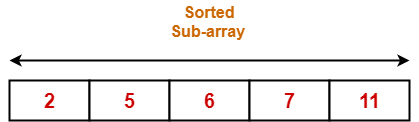With each loop cycle,

• The minimum element in unsorted sub-array is selected.
• It is then placed at the correct location in the sorted sub-array until array A is completely sorted.

## Time Complexity Analysis-

• Selection sort algorithm consists of two nested loops.
• Owing to the two nested loops, it has O(n2) time complexity.

 Time Complexity Best Case n2 Average Case n2 Worst Case n2

## Space Complexity Analysis-

• Selection sort is an in-place algorithm.
• It performs all computation in the original array and no other array is used.
• Hence, the space complexity works out to be O(1).

## Important Notes-

• Selection sort is not a very efficient algorithm when data sets are large.
• This is indicated by the average and worst case complexities.
• Selection sort uses minimum number of swap operations O(n) among all the sorting algorithms.

To gain better understanding about Selection Sort Algorithm,

Watch this Video Lecture

Next Article- Bubble Sort

### Other Popular Sorting Algorithms-

Get more notes and other study material of Design and Analysis of Algorithms.

Watch video lectures by visiting our YouTube channel LearnVidFun.